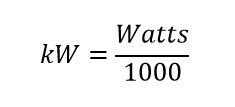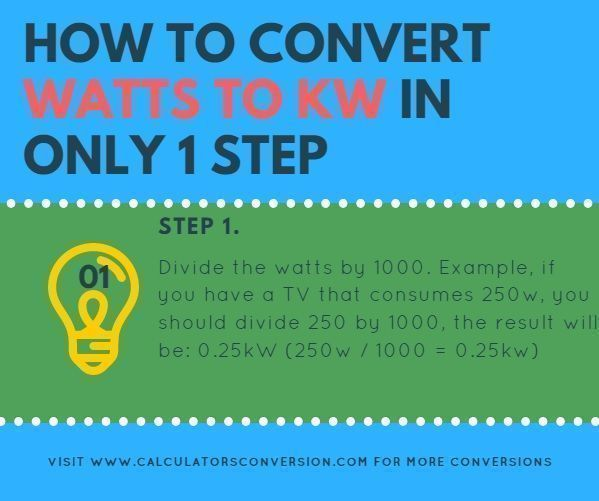# Watts to kW – How to converter automatically, formula and chart (table)

This tool has the ability to automatically convert from Watts to kW, easy, fast and free.

For greater ease we explain that formula is used for the calculation and a table with the main conversions of Watts to kW. Also show how to convert watts to kW in one step

## Watt to kW calculation formula 3phase, 2 phase, 1 phase:• Watts=Watt is the unit of power (symbol: W).
• kW=One kilowatt is equal to 1000 watts.

## How to convert Watt to kW in only 1 step.## Step 1:

Divide the watts by 1000. Example, if you have a TV that consumes 250w, you should divide 250 by 1000, the result will be: 0.25kW (250w / 1000 = 0.25kw)

## Definition watts and kW:

Watts: is Working Power (also called Actual Power or Active Power or Real Power). It is the power that actually powers the equipment and performs useful work.

The real power in watts is the power that performs work or generates heat. Power in watts is the rate at which energy is consumed (or generated). One watt is one joule (energy) per second (1 W = 1 J/s).

Resistive devices or loads such as heaters, incandescent lamps are rated in Watts.

kW: One kilowatt is equal to 1000 watts.

## Watts to kW table chart for conversion, equivalence, transformation:

 Watts kW 1 Watts 0,001 kW 2 Watts 0,002 kW 3 Watts 0,003 kW 4 Watts 0,004 kW 5 Watts 0,005 kW 6 Watts 0,006 kW 7 Watts 0,007 kW 8 Watts 0,008 kW 9 Watts 0,009 kW 10 Watts 0,01 kW 20 Watts 0,02 kW 30 Watts 0,03 kW 40 Watts 0,04 kW 50 Watts 0,05 kW 60 Watts 0,06 kW 70 Watts 0,07 kW 80 Watts 0,08 kW 90 Watts 0,09 kW 100 Watts 0,1 kW 200 Watts 0,2 kW 300 Watts 0,3 kW 400 Watts 0,4 kW 500 Watts 0,5 kW 600 Watts 0,6 kW 700 Watts 0,7 kW 800 Watts 0,8 kW 900 Watts 0,9 kW 1000 Watts 1 kW 2000 Watts 2 kW 3000 Watts 3 kW 4000 Watts 4 kW 5000 Watts 5 kW 6000 Watts 6 kW 7000 Watts 7 kW 8000 Watts 8 kW 9000 Watts 9 kW 10000 Watts 10 kW 20000 Watts 20 kW 30000 Watts 30 kW 40000 Watts 40 kW 50000 Watts 50 kW 60000 Watts 60 kW 70000 Watts 70 kW 80000 Watts 80 kW 90000 Watts 90 kW 100000 Watts 100 kW

Rate this conversion Watts to kW:  [kkstarratings]